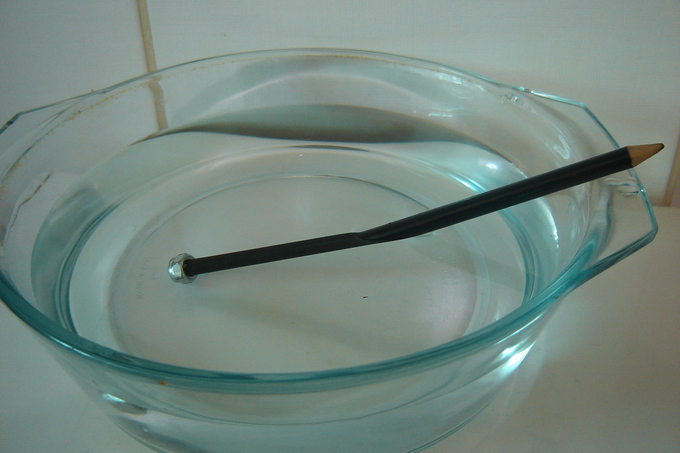# 3.1C: Refraction and Magnification

$$\newcommand{\vecs}{\overset { \rightharpoonup} {\mathbf{#1}} }$$ $$\newcommand{\vecd}{\overset{-\!-\!\rightharpoonup}{\vphantom{a}\smash {#1}}}$$$$\newcommand{\id}{\mathrm{id}}$$ $$\newcommand{\Span}{\mathrm{span}}$$ $$\newcommand{\kernel}{\mathrm{null}\,}$$ $$\newcommand{\range}{\mathrm{range}\,}$$ $$\newcommand{\RealPart}{\mathrm{Re}}$$ $$\newcommand{\ImaginaryPart}{\mathrm{Im}}$$ $$\newcommand{\Argument}{\mathrm{Arg}}$$ $$\newcommand{\norm}{\| #1 \|}$$ $$\newcommand{\inner}{\langle #1, #2 \rangle}$$ $$\newcommand{\Span}{\mathrm{span}}$$ $$\newcommand{\id}{\mathrm{id}}$$ $$\newcommand{\Span}{\mathrm{span}}$$ $$\newcommand{\kernel}{\mathrm{null}\,}$$ $$\newcommand{\range}{\mathrm{range}\,}$$ $$\newcommand{\RealPart}{\mathrm{Re}}$$ $$\newcommand{\ImaginaryPart}{\mathrm{Im}}$$ $$\newcommand{\Argument}{\mathrm{Arg}}$$ $$\newcommand{\norm}{\| #1 \|}$$ $$\newcommand{\inner}{\langle #1, #2 \rangle}$$ $$\newcommand{\Span}{\mathrm{span}}$$$$\newcommand{\AA}{\unicode[.8,0]{x212B}}$$

##### Learning Objectives
• Describe refraction and distinguish between convex and concave lenses

The underlying principle of a microscope is that lenses refract light which allows for magnification. Refraction occurs when light travels through an area of space that has a changing index of refraction. The simplest case of refraction occurs when there is an interface between a uniform medium with an index of refraction and another medium with an index of refraction.Figure: Refraction: As the light is reflected off the pencil we see that, due to the different refraction indexes of water and air, the pencil appears to bend in the water. However, the pencil is straight. It is actually the water acting much like a lens in a microscope that gives it the appearance of bending.

Some media have an index of refraction that varies gradually with position. Therefore, light rays curve through the medium rather than traveling in straight lines. This effect is what is responsible for mirages seen on hot days where the changing index of refraction of the air causes the light rays to bend creating the appearance of specular reflections in the distance (as if on the surface of a pool of water).

Taking advantage of the principle of refraction, devices can be built that can focus light. A device that produces converging or diverging light rays due to refraction is known as a lens. In general, two types of lenses exist: convex lenses, which cause parallel light rays to converge, and concave lenses, which cause parallel light rays to diverge. The former property of convex lenses is of special interest to microbiologists. In essence, a convex lens allows magnification. Light reflecting off an object is focused to a point. The simplest example of this that most people know is a magnifying glass. A magnifying glass is one convex lens, and this by itself allows the magnification of objects.

A microscope is basically a series of lenses that take advantage of the nature of refraction. Due to the nature of light, and the maximum amount of refraction that can be possible by a material, there are limits to the amount of magnification that can be done by a light microscope.Figure: The Microscope: Notice the blue areas, which represent lenses. Note also that many of the lenses are convex, thus the light that goes through a specimen is focused and therefore magnified. Labels: A) Ocular, B) Objective, C) slide holder, D) Illumination Lenses, E) Slide Stage, and F) Illumination Mirror

## Summary

• Convex lenses allow light to converge.
• Concave lenses spread light that travels through it.
• There are limits to the amount of refraction that can be done by a material, and therefore, limits to the amount a microscope can magnify a sample.

## Key Terms

• concave: Curved like the inner surface of a sphere or bowl.
• convex: Curved or bowed outward like the outside of a bowl or sphere or circle.
• specular: Pertaining to mirrors; mirror-like, reflective.

This page titled 3.1C: Refraction and Magnification is shared under a CC BY-SA 4.0 license and was authored, remixed, and/or curated by Boundless.